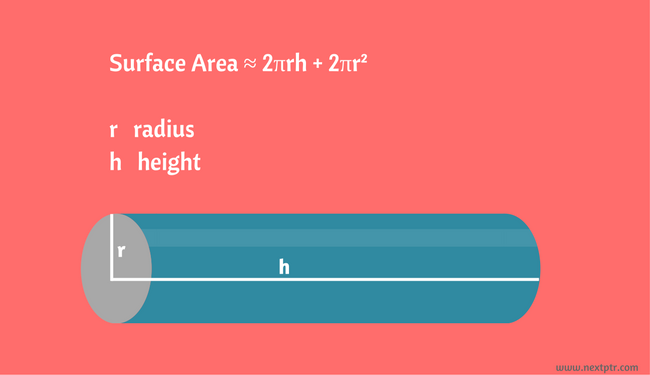# The surface area of a cylinderThe surface area of a cylinder is approximately defined as: 2πrh + 2πr². Where 'r' is the radius and 'h' is the height as shown in the image above.

The cylinder function returns a cylinder object for given radius and height. We create 2 cylinder objects, c1 and c2, and call the surfaceArea method on c1:

``````function cylinder(radius, height) {

var h = height;

return {
surfaceArea: function() {
return 2*Math.PI*r*h + 2*Math.PI*r*r;
}
// ...more methods
};
}

var c1 = cylinder( 5, 100 );
var c2 = cylinder( 5, 200 );

console.log( c1.surfaceArea() );
``````

What will be the approximate value returned by c1.surfaceArea() above?